# Revision questions

Research shows that the best method of revision is to ask yourself questions on a regular basis.
Do you know how to calculate momentum?

An object or group of objects.

An object projected upwards, a moving object hitting an obstacle, an object accelerated by a constant force, a vehicle slowing down and bringing water to the boil in an electric heater.

Kinetic, electrostatic, gravitational potential, magnetic, internal, nuclear, chemical and elastic potential.

Elastic potential energy.

Kinetic energy.

Gravitational potential energy.

Ek = 1/2 x m x v2 (kinetic energy = 1/2 x mass x velocity2).

Ep = m x g x h (gravitational potential energy = mass x gravitational field strength x height).

Joules (J).

Kilograms (kg).

Metres per second (m/s).

Newtons per metre (N/m).

Metres (m).

Metres (m).

Newtons per kilogram (N/kg).

The amount of energy required to raise the temperature of 1kg of a substance by 1oC.

Joules per kilogram per degree Celsius       ( j /kg/oC ).

Degrees Celsius (oC).

Change (delta).

Δθ = final temperature - initial temperature

Measure the mass of a block of material (e.g. copper).  Wrap the block in insulating material and insert a thermometer and heater into the block. Measure the initial temperature of the block. Place an ammeter in series with the heater to measure the current in the circuit. Place a voltmeter in parallel to the power source to calculate the potential difference. Turn the power supply on.  Measure the temperature every minute for ten minutes. Calculate the power supplied using the formula P=VI. Use this to calculate the energy transferred using the formula E=Pt. Plot a graph of energy transferred against temperature. Calculate the gradient of the line. The SHC of the material is calculated by the formula 1/gradient. Repeat the experiment with different materials.

The rate at which energy is transferred.

Watts (W).

P = E / t (Power = energy transferred x time).

P = W / t (Power = work done / time).

Joules (J)

1 Watt = 1 Joule / second

Transferred usefully, stored or dissipated.

Energy cannot be created or destroyed.

Energy is transferred to less useful stores.

Friction and thermal.

Lubrication and thermal insulation.

The higher the thermal conductivity of a material, the higher the rate of energy transfer by conduction.

The thickness and thermal conductivity of the walls.

Cavity wall insulation, loft insulation and double-glazing.

Efficiency = (useful output energy transfer/total input energy transfer) x 100

Efficiency = (useful power output /total power input)

Lubrication and thermal transfer

Oil, coal, gas and uranium.

Solar, wind, waves, hydro-electricity, biofuel, tides and geothermal.

A resource that cannot be renewed and will run out.

A resource that can be replenished after use.

Power production, transport and heating.

Petrol and diesel are used for vehicles. Some vehicles run on biofuels which are renewable.

Natural gas is used for heating in most homes in the UK. Geothermal energy is a renewable energy resource which can be used for heating.

There are no fuel costs and minimal running costs.

Start up costs are high. Electrical production stops when wind speed is too low or too high.

No pollution. Spoils the view. Noisy.

There are no fuel costs and minimal running costs.

High start up costs. A reliable source in sunny countries, but less reliable in cloudy countries. Usually generates electricity on a small scale.

No pollution,

Free, reliable energy.

Not many suitable locations (volcanic area).

No pollution, little damage to the environment.

Immediate electrical production in response to a surge in demand.

High initial set up costs.

No pollution, damage to the environment when building the dam, loss of habitat.

Useful for coastal locations and islands.

Unreliable energy production as waves are reduced when wind drops.

No pollution, disturbs marine habitats, can be a hazard to boats.

No fuel costs and low running costs, a reliable source of energy.

Initial costs are high, limited to estuaries and coastal areas.

No pollution. The damming of estuaries disturbs habitats and prevents access by boats.

Renewable and carbon neutral.

Cost to refine is very high.

Natural habitats cleared to make plantations of biofuels.

No greenhouse gases. Large amounts of energy produced on one site.

Very high start up and running costs.

Radioactive waste needs to be stored for thousands of years before it is safe. Potential for radioactive contamination from waste.

Cost effective production of energy.

Non-renewable.

Produce greenhouse gases and pollution.

Electrical consumption in the UK has fallen.

Fossil fuels and nuclear power.

To reduce greenhouse emissions and become less reliant on non-renewable fuels.

Scientists can give advice to governments about how to tackle environmental issues, but political will is required to enforce legislation that will reduce fossil fuel use.

Local residents complaining about the noise and spoiling the view.

Renewable energy choices are generally more expensive. The location of a home may make it unfeasible to install solar panels (e.g. in a flat).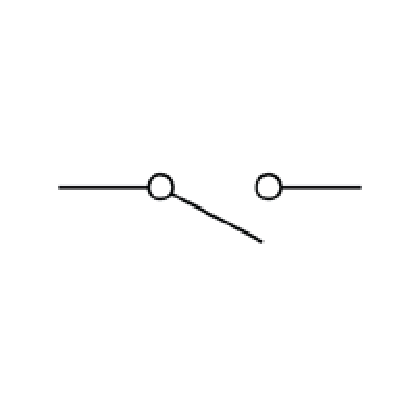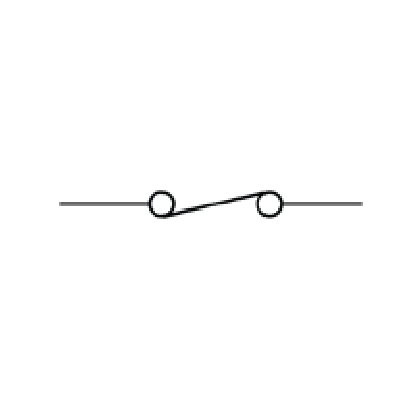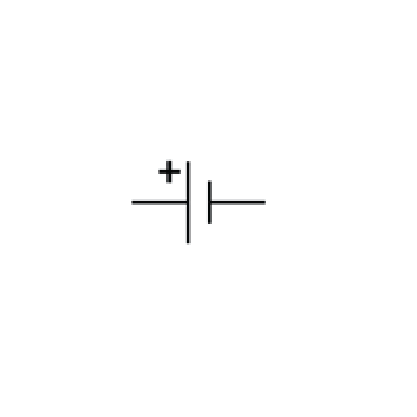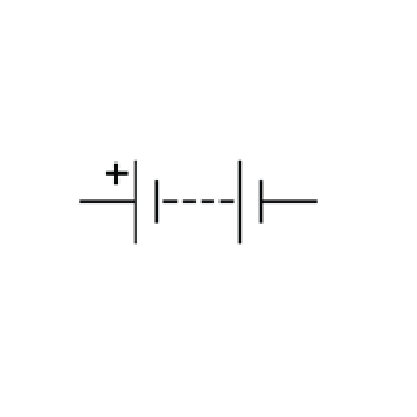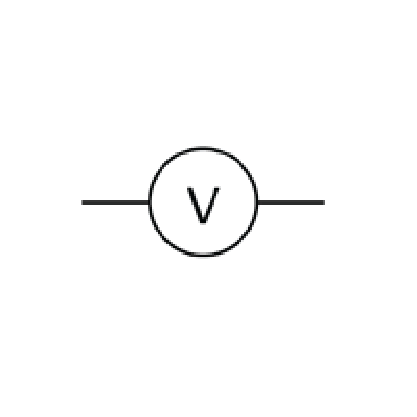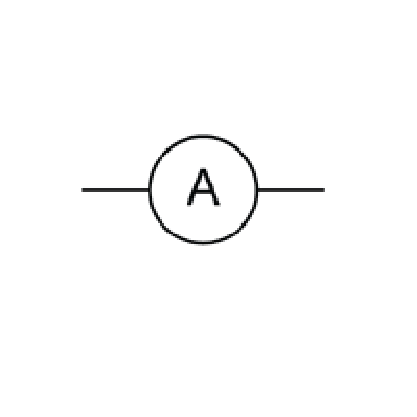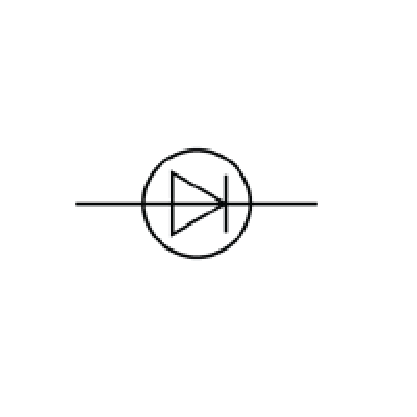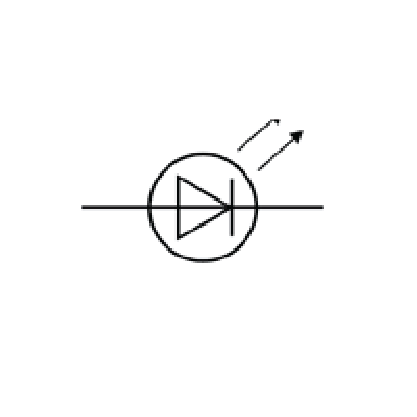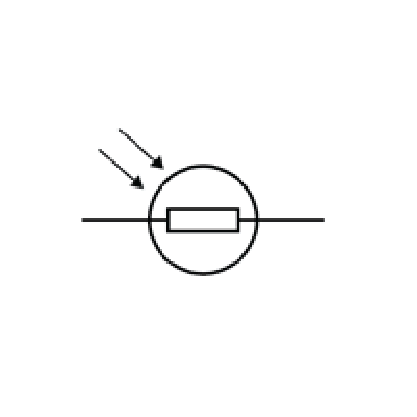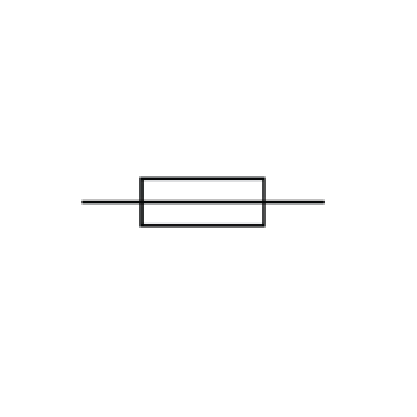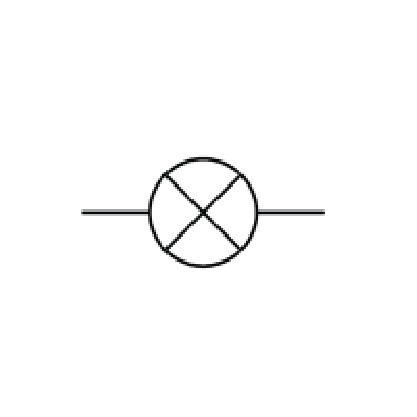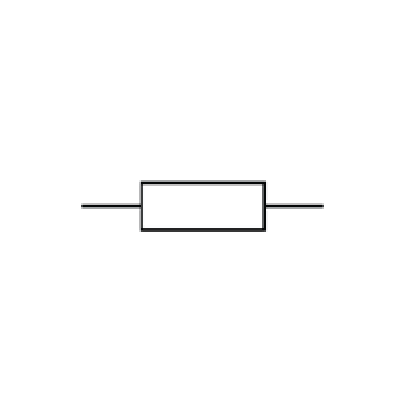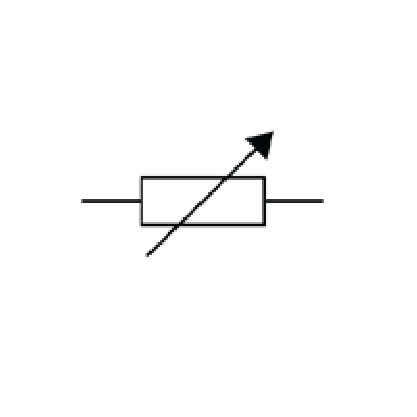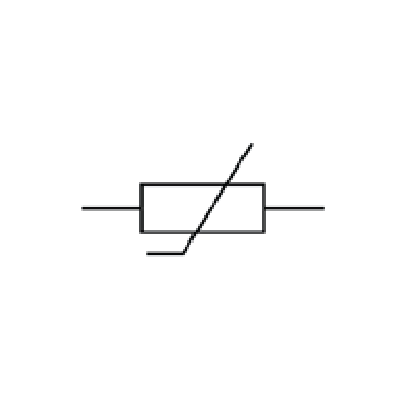The circuit must include a source of potential difference.

A flow of electrical charge.

The rate of flow of electrical charge.

Q = I x t (Charge flow = current x time).

Coulomb (C)

Ampere (amps) (A)

The current has the same value at any point in a single closed loop.

The resistance of the component and the potential difference across the component.

It is a measure of how easy or hard it is for current to flow through a component.

Ohms (Ω)

The greater the resistance the smaller the current.

V = I x R (potential difference = current x resistance)

ohmic conductor

filament lamp

diode

The current is directly proportional to the potential difference. This means the resistance is constant as the current changes.

Current flows through a diode in one direction only. The diode has a very high resistance in the reverse direction.

The resistance of a filament lamp increases as the temperature of the filament increases.

The resistance of a thermistor decreases as the temperature increases.

A thermostat.

The resistance of a LDR decreases as the light intensity increases.

A control circuit to switch on lights when it starts to get dark.

Set up a circuit with an ammeter in series with the component to be tested. Place a voltmeter in parallel to the component. Set the potential difference of the power pack to 2V and measure the current. Repeat for 4V, 6V, 8V and 10V. Turn the component around (to reverse the current). Again set the voltage to 2V on the power pack and record the current. The values on the ammeter and voltmeter will be 0 or negative values.  Calculate the resistance of the component at different voltages using the formula R = V/I. Plot the results on a graph.

A series circuit or parallel circuit.

The components each have the same current.

The total potential difference of the power supply is shared between the components.

The total resistance of two components is the sum of the resistance of each component.

RSum = R1 + R2

The total current is equal to the sum of the currents through the circuit.

The potential difference across each component is the same.

The total resistance of two components is less than the resistance of the smallest individual resistor.

Circuits in parallel, for a given potential difference, have more current for each loop added. Using R = V/I, an increase in current will lead to a decrease in resistance.

AC.

50Hz.

230V.

The current constantly changes direction (50 times per second).

The current flows in a constant direction.

A constant voltage.

A voltage which keeps alternating between positive and negative values.

Live

Neutral

Earth

supply → live wire → fuse → appliance → neutral wire → supply

Fuse and earth wire

Fuse

Earth wire

230V

0V

There is a potential difference in the live wire. If your body provide a bridge between the live wire and earth a current would flow through you.

P = I2R (power = current2 x resistance).

P = V x I (power = voltage x current).

Watts.

Joules.

1 joule per second.

How long the appliance is switched on for and the power of the appliance.

Chemical energy stores in a battery are transferred electrically to the kinetic energy of the motor.

Chemical energy stores (in fossil fuel) are transferred electrically to the internal energy store of the heating element.

Work is done.

E = P x t (energy transferred = power x time).

(E = Q x V) energy transferred = charge flow x potential difference

The power rating indicates the maximum amount of energy transferred per second. The lower the power rating the less energy is used, so the cheaper it is to run.

A system of cables and transformers.

Transformers step up potential difference at the start of the National Grid and step down potential difference at the end of the Grid.

High voltage and low current decreases energy loss along the cables.

25000V

400000V

11000V

230V

Density = mass / volume.

Kg/m3

The behaviour of solids, liquids and gases.

Solids are usually denser than liquids because the particles in a solid are more closely packed. Liquids are usually denser than gases because the particles in a liquid are more closely packed.

Use a ruler to measure the length, width and height of the object. Calculate the volume from these.  Use a balance to calculate the mass. Calculate the density using mass / volume.

Use a balance to measure the mass of the object.  Add 50mL to a measuring cylinder. Place the object in the measuring cylinder. Record the new volume.  Calculate the volume of the object by the difference between the volume of the measuring cylinder before and after. Calculate the density using mass / volume.

Measure the mass of the empty measuring cylinder. Add 50mL of liquid to the measuring cylinder. Measure the mass of the measuring cylinder. Calculate the mass of the liquid using mass of measuring cylinder and liquid - the mass of the empty measuring cylinder. Calculate the density using mass / volume.

Melt, freeze, boil, evaporate, condense and sublimate.

No change in mass.

Physical change.

The energy stored inside a system by the particles that make up the system.

Total kinetic energy + total potential energy of the particles.

Heating changes the energy stored within the system by increasing the energy of the particles that make up the system.

The temperature is raised or there is a change of state.

The increase in temperature depends the mass, the type of material and the energy input into the system.

The amount of energy required to raise the temperature of one kilogram of a the substance by 1oC.

Joules per kilogram per degree Celsius (J/kg/oC).

The energy needed by a substance to change its state.

The energy supplied changes the energy stored (internal energy) but does not change the temperature.

The amount of energy required to change the state of one kilogram of a substance with no change in temperature (e.g. 1kg of water at 100oC becomes 1kg of steam at 100oC).

Joules per kilogram (J/kg).

The energy required to change the state from a solid to a liquid.

The energy required to change the state from a liquid to a vapour (gas).

D

B

A

C

E

Molecules are in constant random motion.

The average kinetic energy of the molecules.

The pressure increases.

The pressure decreases.

As the temperature increases the speed of the molecules increase.  As a result they collide with the sides of the container more often. This results in an increase in the pressure.

1 x 10-10m.

A positively charged nucleus composed of protons and neutrons surrounded by negatively charged electrons.

1 : 10000

The nucleus.

The electrons are found at different distances from the nucleus at different energy levels.

The electrons moves further from the nucleus to a higher energy level.

Moves closer to the nucleus to a lower energy level.

The number of protons is equal to the number of electrons.

They have no overall charge.

Protons.

The number of protons in the atom.

The sum of the protons and neutrons.

electrons = 1, protons = 1, neutrons = 0

electrons = 11, protons = 11, neutrons = 12

electrons = 92, protons = 92, neutrons = 148

Atoms of the same element with different numbers of neutrons.

Ions.

New experimental evidence.

Tiny spheres that could not be divided.

He discovered the electron and suggested the plum pudding model.

Negative electrons embedded in a ball of positive charge (the so called plum pudding model)

Alpha particles were fired at a thin sheet of gold leaf.  The particles where detected using a screen to see how they had been deflected.

Many of the alpha particles passed straight through the gold leaf or were deflected slightly (as expected). However some particles bounced backwards from the gold leaf (not expected)

The mass of the atom was concentrated in a small central nucleus.

He suggested that electrons orbited the nucleus at specific distances or energy levels.

Proton.

Neutron.

The random process of an unstable atom releasing radiation to become more stable.

The rate at which a source of unstable nuclei decays.

Becquerel (bq).

The number of decays recorded each second by a detector.

A Geiger-Muller tube.

Alpha particle (α), beta particle (β), gamma ray (γ) and neutron (n).

Neutron.

Gamma rays.

Alpha particle.

Beta particle.

Alpha particle.

Beta particle.

Alpha particle

Beta particle.

Gamma ray.

Alpha particle.

Alpha particle

Beta particle

They may cause the change in mass and/or charge of the nucleus

Alpha particle emission cause the mass and charge of the of the nucleus to decrease (more negative).

Beta particle emission does not change the mass but does increase the charge of the nucleus (more positive).

Gamma ray emission does not change the mass or charge of the nucleus.

Neutron emission changes the mass but not the charge of a nucleus.Random.

The time it takes for the number of nuclei in a sample to halve, or the time it takes for the activity from an isotope to fall to half its initial level.

It cannot be predicted which radioactive atom in a sample will decay, you can calculate how long it would take for half the sample to decay.

After 3 years 25g remain, after 6 years 12.5g remain, after 9 years 6.25g would remain.

1200Bq decays to 600Bq which decays to 300Bq. Which is 2 half-lives. So one half-life = 5 days (10 days / 2)

12.5%.

2 days

4 years

The unwanted presence of radioactive materials on other materials.

The decaying atoms in the radioactive contamination.

The process of exposing an object to nuclear radiation.

Contamination inside the body is very dangerous. Irradiation is less hazardous because of low penetration.

Less damaging inside the body due to less ionising. Irradiation can be dangerous especially at higher doses.

Contamination carries a low risk but irradiation by gamma rays is highly hazardous.

So the findings can be shared with other scientists and checked by peer review.

A quantity with magnitude only.

A quantity with both magnitude and direction.

Mass, distance, speed and temperature.

Acceleration, force, velocity and displacement.

The direction of the arrow represents the direction of the vector quantity and the length of the arrow represents the magnitude.

An interaction between two objects which are physically touching.

An interaction between two objects which are physically separated.

Friction, air resistance, tension and normal contact force.

Gravitational force, magnetic force and electrostatic force.

Vector

The force acting on an object due to gravity.

The gravitational field around the Earth.

The gravitational field strength where the object is.

W = m x g (weight = mass x gravitational field strength.

N (newtons).

N/kg (newtons per kilogram).

The centre of mass.

They are directly proportional.

Using a calibrated spring-balance (newtonmeter).

A single force that can be used to replace a number forces acting upon an object. The resultant force has the same effect as all the other forces acting together.

Take one force away from the other to calculate the overall resultant force

When a force causes an object to move through a distance (e.g. pushing a pram).

W = F x s (work done = Force x distance)

Joules (J)

When a force of one newton causes a displacement of 1 metre.

1 Joule.

The higher the person gets the greater the gravitational potential energy they store

This causes a rise in the temperature of the object.

Stretching, bending and compression.

If only one force was applied the object would simply move in the direction of the applied force.

This is when an object that has been deformed returns to its original length and shape.

This is when an object that has been deformed does not return to its original length and shape.

The extension of an object is directly proportional to the force applied (provided the limit of proportionality is not exceeded.

This is the point at which the extension is no longer proportional to the force. On a force against extension graph, the line starts to curve after this point.

F = k x e (force = spring constant x extension).

N/m (newtons per metre).

A force that deforms a spring does work. Elastic potential energy is then stored in the spring. The work done is equal to the elastic potential energy stored (unless the spring is inelastically deformed).

A linear relationship shows that the force is directly proportional to the extension.

A non-linear relationship shows the limit of proportionality has been exceeded.

The gradient of the graph (force / extension) is equal to the spring constant.

Ee = 1/2 x k x e2 (elastic potential energy = 1/2 x spring constant x (extension)2

Attach spring to a retort stand. Fix a graduated ruler behind the spring so that the extension can be measured. Measure the starting length of the spring. Add a 0.5N weight (50g) to the spring and measure the extension. Repeat for 1N, 1.5N, 2N, 2.5N, 3N, 3.5N, 4N, 4.5N and 5N. Plot a graph with extension along the horizontal axis and force on the vertical axis. If you get a curve at the top of the graph the limit of proportionality can be calculated.  The spring constant can be calculated from the gradient of the straight line part of the graph.

Distance is how far an object moves.

Scalar.

Displacement is the distance an object moves and the direction it travels.

Vector.

Magnitude and direction e.g. 30m, North.

Scalar

Age, terrain, fitness and distance travelled.

Walking:1.5 m/s, running: 3 m/s, cycling: 6 m/s.

Car: 25m/s, train: 30m/s and aeroplane: 250m/s

330 m/s.

s=v x t (distance travelled = speed x time).

m/s.

The speed of an object in a given direction.

Vector.

An object travelling in s circle is constantly changing direction. As a result its velocity will change constantly due to the change in direction.

Draw a tangent and calculate the gradient of the tangent.

a = Δv / t (acceleration = change in velocity / time taken)

m/s2.

Vector.

Final velocity - initial velocity.

Deceleration (negative acceleration)

V2-U2 = 2 x a x s (final velocity2 - initial velocity2 = 2 x acceleration x distance)

An object falling through a fluid (liquid or gas) will initially accelerate due to gravity. As the velocity increases so will drag as more particles hit the falling object. Eventually the drag will equal velocity and the resultant force will be zero. The object will then move at a constant speed called the terminal velocity.

If the resultant force acting on an object is zero then the object will remain stationary or will continue to move at the same velocity.

When a vehicle is travelled get at a steady speed the resistive forces (air resistance and friction) will be equal to driving force.

The endocrine system is made up of different glands which release chemical messengers called hormones into the blood.

The tendency of objects to remain at rest or continue with uniform motion.

The acceleration of an object is proportional to the resultant force acting upon the object.  It is inversely proportional to the mass of the object.

F = m x a (force = mass x acceleration).

The difficulty of changing the velocity of an object.

The ratio of force over acceleration

~

Set up a trolley attached to a piece of string. Run the string over a pulley at the end of a track.  Set up a light gate to record acceleration. Mark a start line on the track. The distance between the start track and the light gate should be the same each time. Place all the masses in the trolley. Take the first mass and add to the string. Release the trolley from the start line and measure the acceleration. Take the next mass from the trolley and add to the string. Release the trolley and measure the acceleration. Repeat for each of the masses in the trolley (e.g. 50g, 100g, 150g, 200g, 250g). Plot results for force (in newtons) against acceleration.

Whenever two objects interact, the forces they exert on each other are equal and opposite.

The sum of the thinking distance and the braking distance.

The distance travelled during the drivers reaction time.

The distance travelled under braking force.

0.2s to 0.9s

Tiredness, drugs, alcohol and distractions.

Using the drop ruler test, using a digital reaction timer.

Wet and icy.

Worn brakes and worn tyres.

The work done by the friction force between the brakes and the wheel transfers kinetic energy (the cars slows down) to thermal energy in the brakes and tyres (the brakes get hot).

The greater the speed, the greater the braking force required.

The greater the braking force the greater the deceleration of the vehicle.

Overheating of the brakes and loss of control.

V2-U2 = 2 x a x s (final velocity2 - initial velocity2 = 2 x acceleration x distance)

A property of moving objects.

ρ = m x v (momentum = mass xelocity)

Kg m/s (kilogram metres per second)

The total momentum before a collision (or explosion) is equal to the total momentum after a collision (or explosion).What is a closed system? A system where neither energy nor matter can leave.

Calculate the momentum of each each individual vehicle and add them together to get the total momentum before the collision.  Calculate the individual momentum of each of the vehicles after the collision. Use the total momentum before the collision to help calculate an unknown variable after the collision.

A system where neither energy nor matter can leave.

An explosion is a special case where the momentum before the explosion is equal to zero. This means the momentum after the explosion must also equal zero. This is achieved by one of the components (e.g. bullet) having a positive velocity and one of the components (e.g. the rifle) having a negative velocity.

Waves transfer energy from one place to another without transferring matter.

The oscillations are perpendicular to the movement of travel.

The oscillations are parallel to the movement of travel.

Ripples on water, all electromagnetic waves and waves on a string.

Sound waves

An uncompressed section of a longitudinal wave.

An object floating on water will move up and down as a wave passes underneath. Although the wave will move in one direction the floating object does not move with the wave.

Amplitude is the maximum displacement of a point on a wave away from its undisturbed position.

Wavelength is the distance from one point on a wave to the equivalent  point on an adjacent wave.

Frequency is the number of waves that pass a point each second.

Hertz (Hz).

Metres (m).

Seconds (s).

Wave speed is the speed at which the energy is transferred (or the waves move) through the medium.

Wave speed = frequency x wavelength (v = fλ).

Metres per second (m/s).

All waves obey the wave equation.

A

B

Place two microphones 1 metre apart. Attach microphones to a computer with software to detect sound waves.  Start recording and make a sharp and loud sign.  The software will record two distinct peaks. Use the computer to measure the time difference between the sounds detected by each microphone. This is the time it took the sound wave to travel 1 metre.  The speed can be calculated using velocity = distance / time.

Set up a ripple tank. Use a light to project the ripples onto a screen. Use a signal generator to move the dipper. You can count the number of waves that pass a point in 10 secs.  Divide this figure by 10 to calculate the frequency.  Turn on a strobe light. This will make the ripples appear to be still. You can then measure the length of 10 wavelengths. Divide this by 10 to calculate the wavelength. Use the frequency x wavelength to calculate wave speed.

Transverse waves that transfer energy from a source to an absorber.

A continuous spectrum.

They all move at the same velocity

They are grouped by wavelength and frequency.

radio waves - microwaves - infrared - visible light - ultraviolet - x-rays - gamma rays.

gamma rays - x-rays - ultraviolet - visible light - infrared - microwaves - radio waves.

Visible light.

Microwaves transfer energy to the kinetic energy stores of water molecules in the food.

A campfire emits infrared radiation. These are absorbed by objects and transferred to an objects internal energy store.

Substances may absorb, transmit, refract or reflect waves.

The waves transfers energy to the substances internal energy stores.

The wave carries on travelling through the substance.

The wave will change speed as it crosses the boundary between one substance and another.

The wave is neither absorbed or transmitted but its path is changed away from the substance.

Use a Leslie cube with four of the faces coloured with matt black paint, matt white paint, dull metal and shiny metal. Use an infrared thermometer to measure the temperature of a face.  Fill the cube with hot water and place on a heat proof mat.  Place the infrared thermometer at a fixed distance from a face and record the temperature. Repeat for each of the faces. Repeat the experiment to get two sets of results so an average can be calculated.

Radio waves are produced by oscillations in electrical circuits (e.g alternating current).

They create an alternating current with the same frequency as the radio wave itself.

Electromagnetic waves can be absorbed or generated by the atom or nuclei, over a wide frequency range.

Changes in the nuclei of an unstable radioisotope leads to the emission of gamma rays.

Gamma rays, x-rays and ultraviolet light.

The effect depends on the the type of radiation and the size of the dose.

Sievert (Sv)

Ultraviolet light causes skin to age prematurely and increase the risk of skin cancer.

They can cause mutation of genes and cancer.

Satellite communication and cooking food.

Electrical heaters, cooking food and infrared cameras.

Fibre optic communications.

Energy efficient lamps and suntanning.

Medical imaging and treatment.

Long wave signals can be transmitted and recieved without being in line of sight. Short wave signals can be bounced of the atmosphere to travel long distances.

Microwaves used for communication are able to pass through the atmosphere to be picked up by satellites.

Infrared radiation from a heater is absorbed by an object and increases its internal energy stores.

Visible light is refracted which allows it to travel along long lengths of cable. It is not absorbed or scattered as it travels along.

Under normal light the security mark will be invisible to thieves because it is not part of the visible spectrum.

X-rays are transmitted through flesh but are absorbed by denser material such as bone.

These are the places where the magnetic forces are the strongest.

They exert a force on each other.

The magnets repel each other.

The magnets are attracted to each other.

A material that produces its own magnetic field.

A material that becomes a magnet when it is placed in a magnetic field.

Attraction.

It loses most of its magnetism quickly.

Iron, steel, cobalt and nickel.

The region around the magnet where a force acts on magnetic material.

Attraction.

The distance from the magnet.

At the poles.

The direction is given the direction of the force that would act on a north pole placed at that point

From the north pole to the south pole.

A small bar magnet. (The compass needle)

The needle points in the direction of the Earth’s magnetic field.

Move a compass around a magnet and plot the direction of the compass, using arrows, to build up a picture of the magnetic field.

The behaviour of a bar magnet in a magnetic field shows that on Earth they are affect by large magnetic field. As a result a compass can be used to point in a northern direction.

A circular magnetic field is produced around the wire.

The strength of the magnetic field depends upon the current through the wire and the distance from the wire.

A coil of wire.

The magnetic field is strong and uniform.

The magnetic field has a similar shape to a bar magnet.

Adding an iron core to the solenoid.

An electromagnet is a solenoid with an iron core.

When a conductor is placed in a magnetic field, the magnet producing the field and the conductor exert a force on each other called the motor effect.

Direction of motion.

Direction of magnetic field.

Direction of current.

Tesla (T).

B.

Rotate.

One force acts up the other acts down.

Split ring commutator.

Swapping the polarity of the dc supply or swapping the magnetic poles.

Increasing the current, adding more turns to the coil or increasing the magnetic flux density.